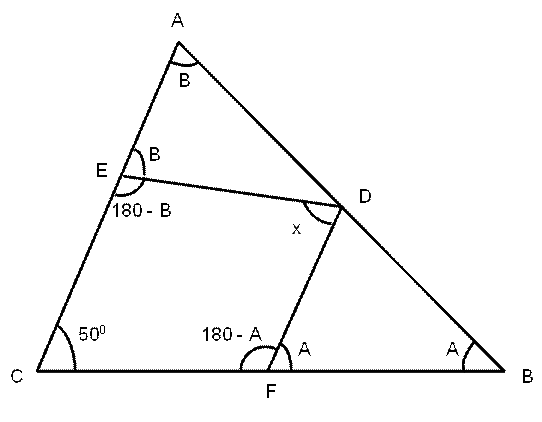# CAT 2021 Question Paper | Quant Slot 3

###### CAT Previous Year Paper | CAT Quant Questions | Question 5

CAT 2021 Quant was dominated by Arithmetic followed by Algebra. In Arithmetic, the questions were dominated by topics like Speed-time-distance, Mixture and Alligations. This year, there was a surprise. The questions from Geometry were relatively on the lower side as compared to the previous years. There were 8 TITA Qs this year. Overall this section was at a medium level of difficulty.

Question 5 : In a triangle ABC , ∠ BCA =50°. D and E are points on AB and AC, respectively, such that AD = DE. If F is a point on BC such that BD = DF, then ∠ FDE, in degrees, is equal to

1. 100
2. 80
3. 96
4. 72

## Best CAT Coaching in Chennai

#### CAT Coaching in Chennai - CAT 2022Limited Seats Available - Register Now!

We draw the diagram carefully with the given information.From the triangle ABC,
∠A + ∠B + ∠C = 1800
∠A + ∠B + 500 = 1800
∠A + ∠B = 1300
∠C + ∠F + ∠D + ∠E = 3600
500 + 1800 - ∠A + ∠x + 1800 - ∠B = 3600
500 + ∠x = ∠A + ∠B
500 + ∠x = 1300
∠x = 800
∠FDE = 800

The question is " In a triangle ABC , ∠ BCA =50°. D and E are points on AB and AC, respectively, such that AD = DE. If F is a point on BC such that BD = DF, then ∠ FDE, in degrees, is equal to "

##### Hence, the answer is '80'

Choice B is the correct answer.

###### CAT Coaching in ChennaiCAT 2023

Classroom Batches Starting Now! @Gopalapuram

###### Best CAT Coaching in Chennai Introductory offer of 5000/-

Attend a Demo Class

##### Where is 2IIM located?

2IIM Online CAT Coaching
A Fermat Education Initiative,
58/16, Indira Gandhi Street,
Kaveri Rangan Nagar, Saligramam, Chennai 600 093

##### How to reach 2IIM?

Mobile: (91) 99626 48484 / 94459 38484
WhatsApp: WhatsApp Now
Email: info@2iim.com